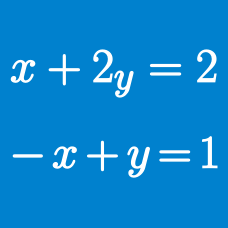Algebra

# System of Equations - Substitution

If $x$ and $y$ are non-zero real numbers satisfying the system of equations

$\begin{cases} x-y= 24, \\ x^2-2xy-y=24, \end{cases}$ what is the value of $x+y$?

Let $a$ and $b$ be the values of positive integers $x$ and $y$, respectively, that satisfy $x-y=5, x^2+y^2=73.$ What is the value of $5a+8b?$

If there exists exactly one solution $(x, y)$ that satisfies the simultaneous equations $x-14y=-1, x^2+xy+m=0,$ what is the value of $\frac{1}{m}$?

For the simultaneous equations $x+y = 2k+16, xy+x+y = k^2+5k+16$ to have real solutions, it must be the case that $k \geq -\frac{a}{b}$, where $a$ and $b$ are coprime positive integers. What is the value of $a+b$?

For what value of $k$ does there exist exactly one pair $(x, y)$ that satisfies the simultaneous equations $y-14x=k, x^2+y=5?$

×